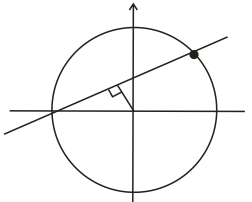Q

# Solve this problem - Co-ordinate geometry - JEE Main-6

The sum of the squares of the lengths of the chords intercepted on the circle, , by the lines where is the set of all natural numbers, is :

• Option 1)

• Option 2)

• Option 3)

• Option 4)

Viewsthen length of perpendicular from centre to line

length of intercepts =

Possible value of n are =

Sum of squares of length =

Option 1)

Option 2)

Option 3)

Option 4)

Exams
Articles
Questions